• English
• Account
• API
• Integrations
• Enterprise
• Resources
The essentials of Airtable formulas
• 12 Sep 2023
• Dark
Light

# The essentials of Airtable formulas

• Dark
Light

Article Summary

In Airtable there are many different fields that you can add to a table; one of them is the formula field type. Formulas let you reference other fields in a table and create different functions based on the content of those fields. This article will cover how to write and format the formula field type.

## Creating a formula field type

To write a formula, you first need to configure a new field as a formula field type.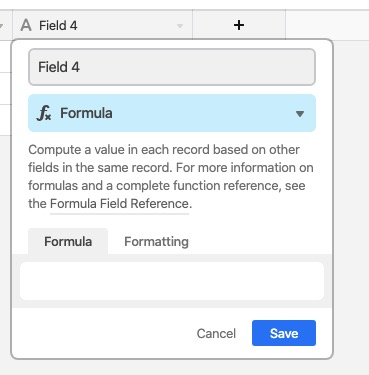## How to write a formula

Once you create a formula field, you can start writing the formula directly in the field's text box.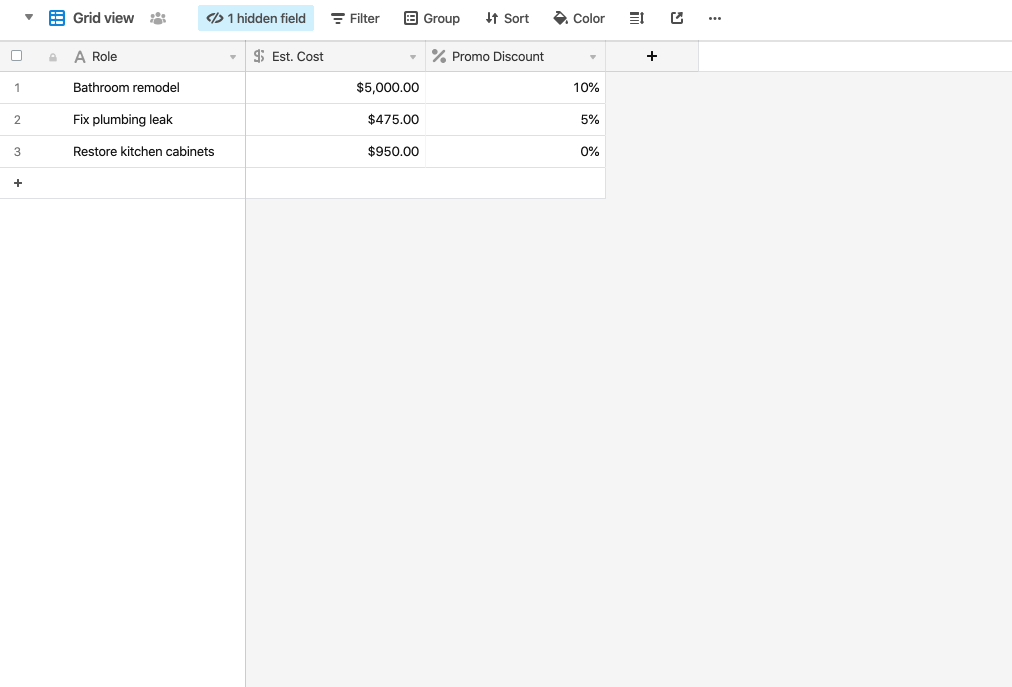## Formatting the result of a formula

Every formula results in some result - either a number, a date, or text - and how you can apply formatting to that result varies.

### Number format

When the output of a formula is a number, you can format it by double clicking the field name, selecting customize field type, and then selecting formatting. Then, you can apply the following options to the output of your formula:

• Decimal
• Integer
• Currency
• Percent
• Duration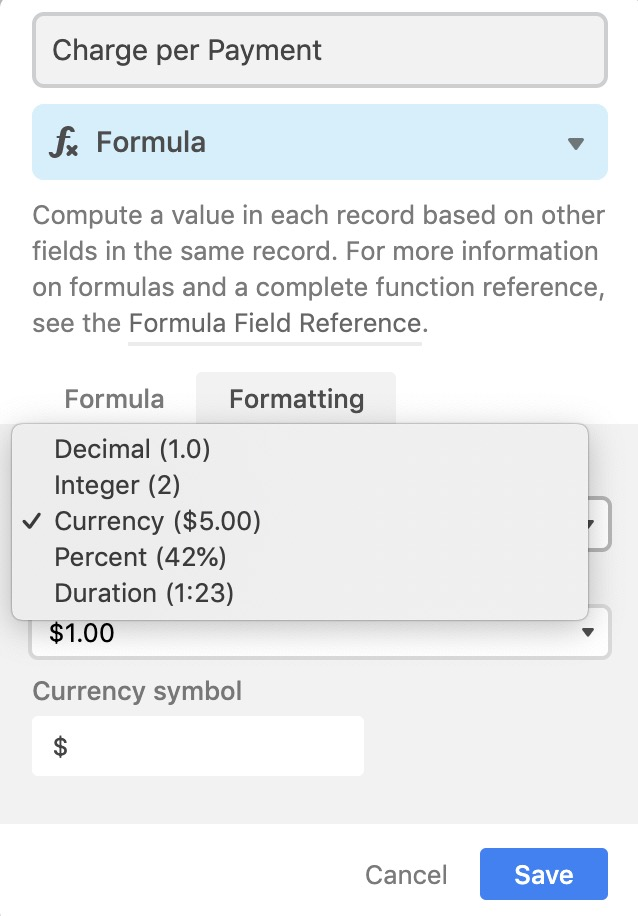### Date format

When the output of a formula is a date, you can apply the following options to the output of your formula:

• Date format (local, friendly, US, European, ISO)
• Include a time field
• Use the same time zone (GMT) for all collaborators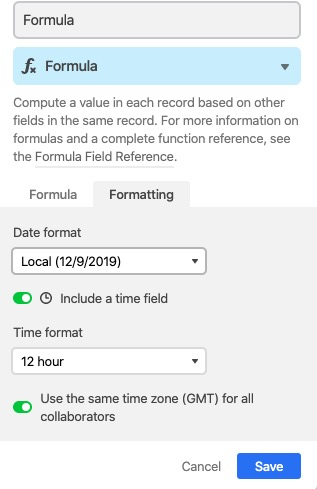### Text

When the output of a formula is text, that means that it potentially contains a mix of dates, numbers, and/or other plain text. Since there isn't a single way to format this information, you'll see a note in the formatting tab to notify you.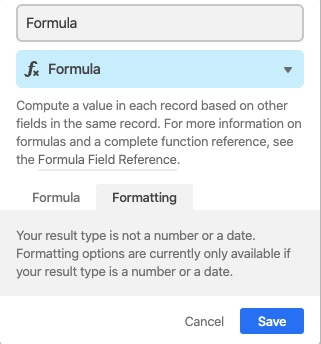TIP